﻿ Flat Space Cosmology as an Alternative to Lcdm Cosmology

### Flat Space Cosmology as an Alternative to Lcdm Cosmology

Eugene Terry Tatum, U.V.S. Seshavatharam, S. Lakshminarayana

Frontiers of Astronomy, Astrophysics and Cosmology

## Flat Space Cosmology as an Alternative to Lcdm Cosmology

Eugene Terry Tatum1,, U.V.S. Seshavatharam2, S. Lakshminarayana3

1760 Campbell Ln. Ste. 106 #161, Bowling Green, KY 42104

2Honorary faculty, I-SERVE, Alakapuri,Hyderabad-35, Telangana, India

3Dept. of Nuclear Physics, Andhra University, Visakhapatnam-03, AP, India

### Abstract

Flat space cosmology is a recently introduced cosmological model which appears to be a useful heuristic model for correlating current astronomical observations. Based upon a few number of reasonable initial assumptions, our cosmological model universe is always flat and yet it shows a remarkably robust inflationary expansion effect within a tiny fraction of the first second of universal time. Our thermodynamic formula closely resembles Hawking’s black hole temperature formula, and yet it appears to correlate closely with cosmic temperature vs. time derivations from experiments in particle physics. Lastly, our model supports recent credible challenges to the current concept of dark energy.

• Eugene Terry Tatum, U.V.S. Seshavatharam, S. Lakshminarayana. Flat Space Cosmology as an Alternative to Lcdm Cosmology. Frontiers of Astronomy, Astrophysics and Cosmology. Vol. 1, No. 2, 2015, pp 98-104. http://pubs.sciepub.com/faac/1/2/3
• Tatum, Eugene Terry, U.V.S. Seshavatharam, and S. Lakshminarayana. "Flat Space Cosmology as an Alternative to Lcdm Cosmology." Frontiers of Astronomy, Astrophysics and Cosmology 1.2 (2015): 98-104.
• Tatum, E. T. , Seshavatharam, U. , & Lakshminarayana, S. (2015). Flat Space Cosmology as an Alternative to Lcdm Cosmology. Frontiers of Astronomy, Astrophysics and Cosmology, 1(2), 98-104.
• Tatum, Eugene Terry, U.V.S. Seshavatharam, and S. Lakshminarayana. "Flat Space Cosmology as an Alternative to Lcdm Cosmology." Frontiers of Astronomy, Astrophysics and Cosmology 1, no. 2 (2015): 98-104.

 Import into BibTeX Import into EndNote Import into RefMan Import into RefWorks

1
Prev Next

### 1. Introduction

Standard LCDM cosmology is currently widely accepted as the “gold standard” of Big Bang cosmology . The LCDM model incorporates a theory of “cosmic inflation”, as well as the concepts of “cold dark matter” and “dark energy”. We have recently introduced our competing alternative heuristic cosmological model for correlating current astronomical observations [2, 3]. Because our model is always at the Friedmann critical average mass density it is always “flat” by this definition; hence, the name “flat space cosmology”.

In reviewing our first introductory paper , the reader will see how knowing only the current cosmic microwave background radiation (CMBR) temperature of 2.725 K allows one to derive credible current values for the Hubble parameter, Hubble radius, Hubble time and total mass of our observable universe. In our second introductory paper , we derived a thermodynamic formula closely resembling Hawking’s black hole temperature formula and closely correlated the CMBR redshift of 1093 with the recombination temperature of 3,000 K. This has given us some confidence in the reliability of flat space cosmology as a useful heuristic model.

### 2. Basic Assumptions of Flat Space Cosmology

Our key model assumptions can be expressed as follows, for any scale starting from the Planck scale to the scale of our observable universe:

1. Cosmic radius R and total mass MR follow the Schwarzschild formula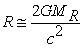at all times.

2. The cosmic horizon always translates at speed of light c with respect to its geometric center. Thus, in our model, the Hubble parameter HR can be expressed as c/R. And considering assumptions 1 and 2 together, the cosmic Hubble radius can be expressed as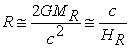and Hubble time (universal age) can be expressed as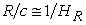for any stage of cosmic expansion.

3. Following thermodynamics of Hawking’s black hole temperature formula , at any radius R cosmic temperature TR is inversely proportional to the geometric mean of cosmic total mass MR and the Planck mass MPl.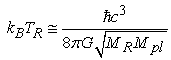(1)

Comment: Although our first introductory paper also incorporated a cosmic rotation assumption, recent analysis of conservation of angular momentum within our model has lead us to conclude that our model universe can have, at most, an angular momentum of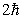kg.m2sec-1 and a current angular velocity of 10-139 rad.sec-1, which would be entirely undetectable by any technology we can imagine.

### 3. Model Equations for Flat Space Cosmology

A. Relations between cosmic radius, total mass and Hubble parameter: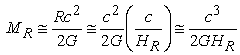(2)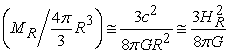(3)

where R, MR and HR represent cosmic radius, total mass and Hubble parameter, respectively. Average mass density equaling Friedmann critical density is seen in relation (3). Hence, our cosmic model is constantly “flat” as defined by the Friedmann critical density formula.

B. Relations between temperature, mass, radius and Hubble parameter (thermodynamics):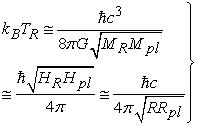(4)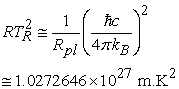(5)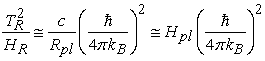(6)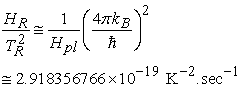(7)

where TR represents the cosmic temperature for a given cosmic radius R and RPl is the Schwarzschild radius corresponding to Planck mass MPl.

### 4. Our Derivations of Current Cosmological Parameters

Using only our basic assumptions and the equations they generate above, derivations of current values for our observable universe are as follows:

Relations between universal current radius R0, current temperature T0, current Hubble parameter H0, current total mass M0, and current average mass-energy density: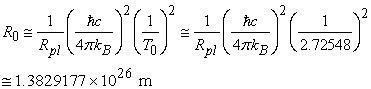(8)(9)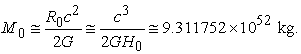(10)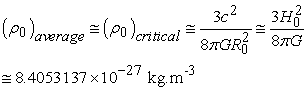(11)

where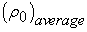is the average mass density and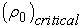is Friedmann’s critical average mass density.

The above-derived radius and mass values simulate a current observable universe with a radius of 14.6 billion light-years and roughly 2 x 1022 visible stars plus 5x dark matter, or roughly 1053 kg.

Our analysis of conservation of angular momentum in flat space cosmology (pending publication), indicates the maximum possible Planck scale angular momentum to be: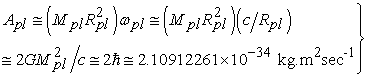(12)

where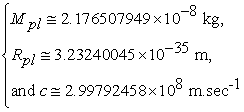Assuming angular momentum conservation, maximum possible current angular momentum is: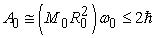(13)

Hence, the maximum possible current angular velocity is: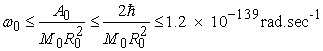(14)

Based upon this extremely small derived maximum theoretical value, our pending angular momentum paper concludes “this number is well beyond our ability to observe cosmic rotation effects in the present” or, presumably, even at the universal age at recombination. Thus, our model predicts that rotational effects are unlikely to be detectable in higher resolutions of the cosmic microwave background radiation (CMBR).

As our cosmic model is always assumed to be expanding at light speed, from the beginning of the Planck scale, cosmic age can be expressed as follows: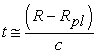(15)

For the current case, since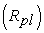is very small and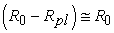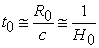(16)

### 5. Our Model Correlation with Observed CMBR Redshift

We have proposed simple model equations (relations 17-20) for observed and predicted cosmic redshifts , including the CMBR redshift. One particularly simple model equation under current study is: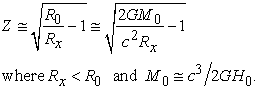(17)

and where R0 and Rx represent current and past cosmic radii, respectively. With reference to the assumed equivalent cosmic temperature from our second introductory paper , redshift term Z can be obtained in the following way: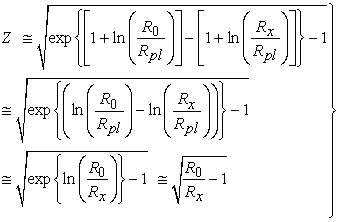(18)

From relation (5) it is clear that the cosmic radius is inversely proportional to the squared cosmic temperature. The above relation (17) can be expressed, approximately, as follows: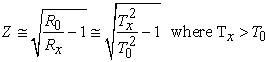(19)

For past higher cosmic temperatures,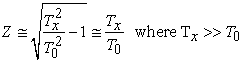(20)

This can be compared with the famous relation familiar to modern cosmologists: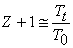(21)

The following graph (Figure 1), according to above relation (17), shows expected cosmic redshift as a function of the log ratio of current cosmic radius (R0) to past cosmic radius (Rx) pertaining to a particular astronomical observation. In this manner, increasingly greater redshifts would be expected to correspond with more distant galactic observations. However, notice the apparent near-linearity below the radius ratio of about 20 (Log 1.3), and the increasingly nonlinear appearance with deeper space observations. The authors propose that something like this mathematical relationship could be useful in modeling the results of progressively deeper space observations. For data, see Table 1. The last row of Table 1 (next page) correlates cosmic radius (Rx), Log (Rx/R0), redshift and universal age corresponding to a temperature of 3116 K.

#### Table 1. Cosmic Physical Parameters Obtained with Above RelationsDownload asPowerPoint Slide

Veiw figure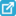View current table in a new windowDownload asVeiw figureFigures index
Figure 1. Cosmic Redshift vs Log Ratio of Current and Past Cosmic Radii

Thus, simple model formula relations (17) and (20) closely approximate the recombination temperature of 3000 K and CMBR redshift of 1093 believed to be related to formation of the first hydrogen atoms.

The following figure (Figure 2) is a summary log graph showing how our model equations tightly correlate universal age (Hubble time), Hubble radius, total mass M0 and cosmic temperature. It is noteworthy that this graph shows a pronounced cosmic inflation effect for the early universe .In particular, the Hubble radius in meters expands by 25 logs of 10 within the first 10-17second. The significance of this is addressed in the discussion section below.Download asVeiw figureFigures index
Figure 2.

The tight correlation between universal age and cosmic temperature in our model is perhaps more noticeable when we overlay the same summary log graph with a color-coded map derived from theoretical and experimental particle physics. The color code corresponds to the various particle physics epochs of the early universe. The universal age and temperature correlations are fairly well worked out for temperatures below about 1015 K, because they can be closely simulated in our most energetic particle accelerators, including the Large Hadron Collider at CERN. However, they remain purely theoretical at temperatures higher than about 1015 K.Download asVeiw figureFigures index
Figure 3.

### 6. Discussion

Since the initial development of the Big Bang theory in the early and mid-20th century, there have been three major refinements to the theory. In chronological order, these refinements (largely necessitated by astronomical observations) include the very credible evidence of dark matter , the current theory of cosmic inflation (“new inflation”) [6, 7], and the 1998 Type Ia supernovae data  purporting to show evidence of a positive cosmological constant (“dark energy”). The most widely accepted Big Bang cosmological model incorporating these refinements is usually referred to as LCDM cosmology.

LCDM cosmology is one the few remaining cosmological models consistent with the recently released 2015 Planck survey data. The Planck survey data appear to eliminate all noninflationary models. Perhaps most importantly, the Planck survey data show very strong evidence that our currently observable universe is extremely flat. Any remaining cosmological model in competition with LCDM cosmology must explain the extreme flatness of our universe. The only models which can do this are of two major types: those which are always flat (such as our model); and those which just happen to be flat at the present time, although they may have significant positive or negative curvature at other stages of universal expansion.

The LCDM cosmology model has sufficiently robust inflation in the very early universe that even a highly curved early universe would be expected to be flat at the present time. However, LCDM cosmology also incorporates a dark energy dominated final stage of progressively more negative curvature.

Of late, there have been several critical commentaries on the theory of cosmic inflation, most notable among which is from one of its creators, Dr. Paul Steinberg . One reasonable criticism of the theory is that it seems to imply an infinite number of parallel universes in which every possible permutation not only happens, but happens an infinite number of times! As Dr. Steinhardt has so rightly pointed out, a theory which predicts every conceivable outcome predicts nothing.

We would also point out that a model in which inflation turns on and then off and then on again (in the final form of dark energy), and yet gives us a perfectly flat universe at the present time, appears to violate the Copernican principle, where we just happen to be living at the time when the attraction of gravity and the repulsion of dark energy appear to be in perfect balance.

The current theory of cosmic inflation operates on several assumptions. Considering that large-scale homogeneity was assumed in the development of general relativity (Einstein assumed an infinite universe), it had been a puzzle to cosmologists as to how a universe assumed to be much larger than our own Hubble radius could have had any kind of causal connection to generate homogeneity. This has been called the “horizon problem.” The theory of cosmic inflation assumes such large-scale homogeneity and incorporates an extremely brief period of superluminal hyper-rapid exponential expansion which simultaneously solves the flatness problem and the horizon problem .

Our cosmic model has a different set of reasonable assumptions. We don’t automatically assume our universe to be considerably larger beyond what we can now see. We don’t automatically assume that space-time “stretches” at superluminal speed. General relativity works just as well with a finite flat universe of 14.6 billion light-years in radius as it does with a universe of infinite proportions. A crucial difference, however, is that our model universe is confined to a size limited by its speed of light Hubble radius. This solution to the “horizon problem” is built into our model, not because we designed it with that intention, but because a universe bounded by a speed of light Hubble radius should to be causally connected.

The theory of cosmic inflation also postulates that primordial density perturbations resulting from primordial quantum fluctuations are responsible for the structure of the universe we see today. This also seems reasonable in “flat space cosmology.”

Our flat space cosmology model, perhaps surprisingly, also shows pronounced hyper-rapid inflationary expansion of the early universe (25 logs of 10 within the first 10-17 second in our Figure 2 summary log graph), but without the attendant problems at the heart of the theory of cosmic inflation as reported by Dr. Steinhardt . In contrast to the abrupt stops and starts and temporary flatness of new inflation, our cosmic model is constantly flat and never turns off the expansion process.

In fact, in contrast to the classic textbook model of a flat universe constantly decelerating due to attractive gravity but never quite coming to a complete stop, our cosmic horizon just keeps coasting along at speed of light c. We postulate that this effect may be what has been mistaken for dark energy, since our most distant observable galaxies are clearly not decelerating. The reader will, of course, object that dark energy is, in fact, causing cosmic acceleration. However, there are recent credible arguments that the 1998 Type Ia supernovae data are not at all conclusive in this regard. Please see references [11, 12, 13]. In fact, a very strong case has been made by Jun-Jie Wei, et al., that the Type Ia supernovae data are a better fit for a cosmic horizon coasting along at speed of light c !

So, what would it imply if our observable universe has not undergone superluminal expansion and is not subject to a mysterious new type of “energy”? First of all, it would imply that the assumed volume of our observable universe has been grossly overestimated by at least a factor of ten. Secondly, it would imply that there is no mass-energy component of the calculated critical mass density attributable to dark energy. This would then imply that our calculated M0 value (the baryonic matter plus the approximately 5x cold dark matter) from relation (10) may be all one needs to divide by our Hubble volume to determine the average mass density of our universe. We would then observe by relation (11) that the calculated average mass density of our Hubble volume is 8.4 x 10-27 kg.m-3, which is exactly what has long been predicted for our universe.

### 7. Conclusions

In this paper, we have attempted to show that flat space cosmology is a viable, and perhaps superior, alternative to standard LCDM cosmology. Our introductory papers [2, 3] have clearly demonstrated that, with very few basic and reasonable model assumptions, a variety of astronomical observations can be correlated to a remarkable degree. The reader is referred to the summary log graphs (Figures 2 and 3) to see how the model equations may correlate all the way down to the Planck scale and to the cosmic temperature vs universal age predictions from theoretical and experimental particle physics.

In the discussion section we have pointed out some of the most important objections to the theory of cosmic inflation as currently formulated, not the least of which are the abrupt transitions, implication of infinitely many parallel universes, and the apparent violation of the Copernican principle. We have demonstrated in the summary log graphs that our own model exhibits a robust period of hyper-rapid exponential expansion (25 logs of 10 in the first 10-17 second). However, in contrast to the theory of cosmic inflation, the naturally evolving inflationary expansion of our model shows no abrupt transitions and continues without slowing down (unlike the constantly decelerating textbook flat universe).

In the final part of the discussion section, after dispelling the unreasonable notion of superluminal expansion, and referencing the marginal evidence for cosmic acceleration [11, 12, 13], we explain why our derived total mass M0 (baryonic mass plus 5x dark matter) divided by the Hubble volume gives us the long-predicted universal average mass density of 8.4 x 10-27 kg.m-3. We humbly invite the reader to explore this fascinating subject [14, 15, 16, 17, 18]!

### Acknowledgements

The authors express their thanks to Dr. Abhas Mitra for his kind and valuable suggestions in developing this subject. One of the authors, Seshavatharam U.V.S, is indebted to professors K.V. Krishna Murthy, Chairman, Institute of Scientific Research in Vedas (I-SERVE), Hyderabad, India and Shri K.V.R.S. Murthy, former scientist IICT (CSIR), Govt. of India, Director, Research and Development, I-SERVE, for their valuable guidance and great support in developing this subject. Author Dr. E. Terry Tatum would also like to thank Dr. Rudy Schild, Harvard Center for Astrophysics, for his support and encouragement in developing this subject.

### References

  Planck Collaboration: Planck 2015 results. XIII. Cosmological parameters. http://arxiv.org/abs/1502.01589. (2015).In article  Tatum, E.T., U.V.S. Seshavatharam and S. Lakshminarayana. The Basics of Flat Space Cosmology.International Journal of Astronomy and Astrophysics, 5: 116-124. (2015).In article View Article  Tatum, E.T., U.V.S. Seshavatharam and S. Lakshminarayana. Thermal Radiation Redshift in Flat Space Cosmology.Journal of Applied Physical Science International.4(1): 18-26 (2015).In article  Hawking, S.W. Particle Creation by Black Holes. Commun.Math. Phys., 43: 199-220. (1975).In article View Article  Rubin, V.C. and Ford, W.K., Jr. Rotation of the Andromeda Nebula from a Spectroscopic Survey of Emission Regions. The Astrophysical Journal, 159: 379-403.In article View Article  Linde, A.D. A New Inflationary Universe Scenario: A Possible Solution of the Horizon, Flatness, Homogeneity, Isotropy, and Primordial Monopole Problems. Physics Letters, 108B: 389-92. (1982).In article View Article  Albrecht, A. and Steinhardt, P.J. Cosmology for Grand Unified Theories with Radiatively Induced Symmetry Breaking. Physical Review Letters, 48: 1220-3. (1982).In article View Article  Riess, A.G., et al. Observational Evidence from Supernovae for an Accelerating Universe and a Cosmological Constant. stronomical J., 116 (3): 1009-38. (1998).In article  Steinhardt, P.J. The Inflation Debate: Is the Theory at the Heart of Modern Cosmology Deeply Flawed? Scientific American, 304(4) pp. 18-25. (2011).In article View Article  Guth, A.H. Inflationary Universe: A Possible Solution to the Horizon and Flatness Problems. Phys. Rev., D23: 347. (1981).In article View Article  Nielsen, J.T. et al. Marginal Evidence for Cosmic Acceleration from Type Ia Supernovae. arXiv:1506.01354v1. (2015).In article  Jun-Jie Wei, et al. A Comparative Analysis of the Supernova Legacy Survey Sample with ΛCDM and the Rh=ct Universe. The Astronomical Journal, 149: 102. (2015).In article View Article  Mitra, A. Energy of Einstein's Static Universe and its Implications for the Λ CDM Cosmology. JCAP, 03: 007. (2013).In article  Tatum, E.T. Could Our Universe Have Features of a Giant Black Hole? Journal of Cosmology, 25: 13061-13080. (2015).In article  Tatum, E.T. How a Black Hole Universe Theory Might Resolve Some Cosmological Conundrums. Journal of Cosmology, 25: 13081-13111. (2015).In article  U.V.S. Seshavatharam, and S. Lakshminarayana, Primordial Hot Evolving Black Holes and the Evolved Primordial Cold Black Hole Universe.Frontiers of Astronomy, Astrophysics and Cosmology, 1(1): 16-23. (2015).In article  U.V.S. Seshavatharam, S. Lakshminarayana. Friedmann cosmology: Reconsideration and New Results. International Journal of Astronomy, Astrophysics and Space Science, 1(2):16-26. (2014).In article  Pathria, R.K. The Universe as a Black Hole. Nature 240 (5379):298299. (1972).In article View Article